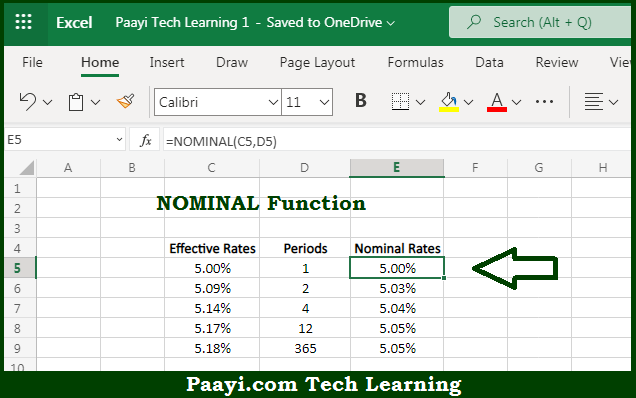# Learn How to Use Microsoft Excel NOMINAL Function

Written by | 0 Comments | 606 Views

In this article, you will learn how to use the Microsoft Excel NOMINAL function and its prime function in Microsoft Excel. You will also get to know the Microsoft Excel NOMINAL function return value and syntax with the help of some examples.

Microsoft Excel NOMINAL Function

The main purpose of the Microsoft Excel NOMINAL function is to get the nominal annual interest rate. That implies, with the help of the NOMINAL function you can able to return the nominal interest rate when given as an effective annual interest rate and the number of compounding periods per year. It should be noted that the effective rate is the actual rate due to compounding. Also, the nominal rate is usually the stated rate. So, with the help of the NOMINAL function, you can able to get the nominal annual interest rate.

Return Value of NOMINAL Function

The return value will be the nominal interest rate.

Syntax of NOMINAL Function

=NOMINAL(effect-rate, npery)

Where the arguments:

• effect-rate: This is the effective annual interest rate.
• npery: This is the number of compounding periods per year.

## How to Use Microsoft Excel NOMINAL Function?So we know that Microsoft Excel NOMINAL function you can able to get the nominal annual interest rate. That implies, with the help of the NOMINAL function you can able to return the nominal interest rate when given as an effective annual interest rate and the number of compounding periods per year. It should be noted that the effective rate is the actual rate due to compounding. Also, the nominal rate is usually the stated rate. So, with the help of the NOMINAL function, you can able to get the nominal annual interest rate.